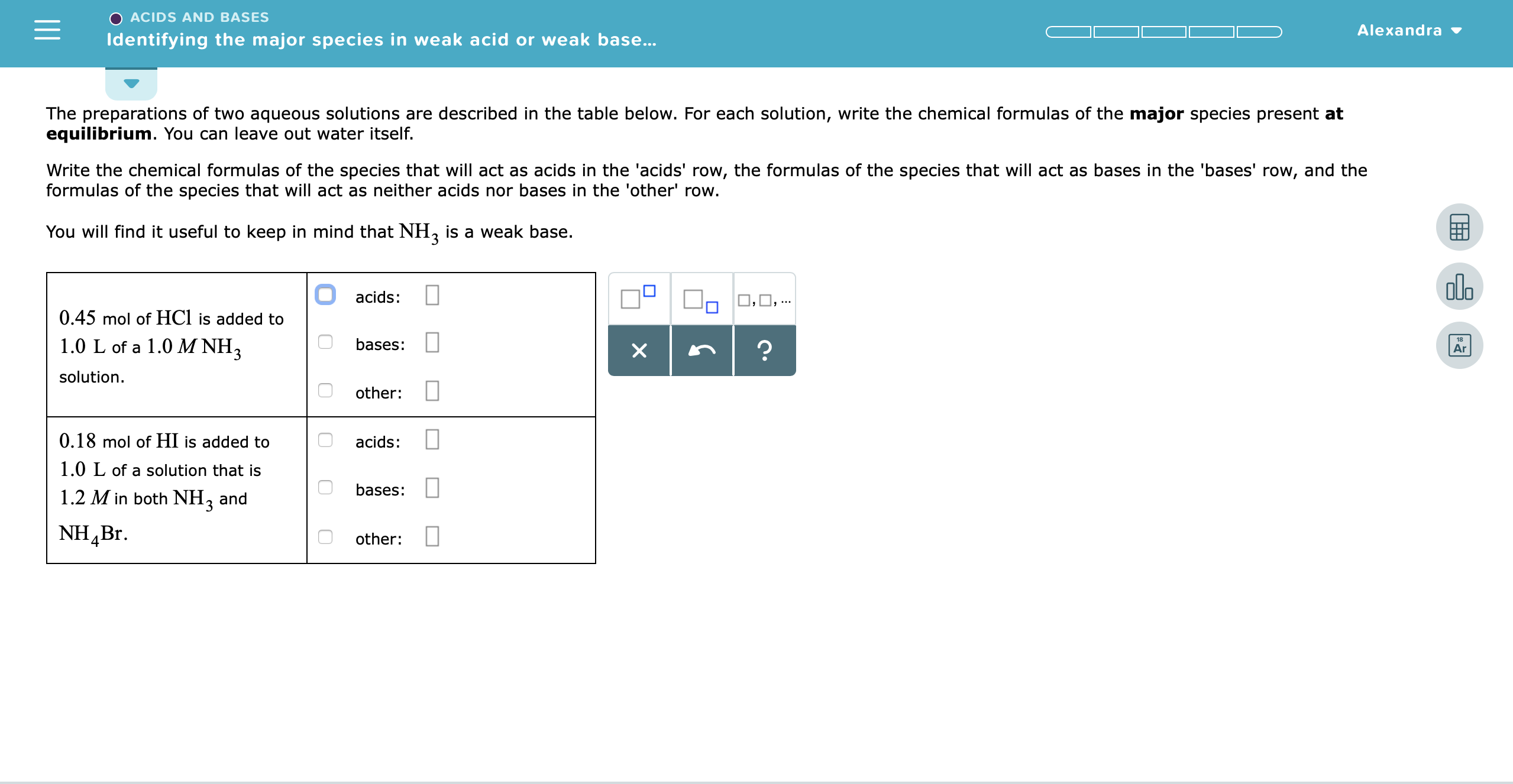# The preparations of two aqueous solutions are described in the table below. For each solution, write the chemical formulas of the major species present equilibrium. You can leave out water itself. at Write the chemical formulas of the species that will act as acids in the 'acids' row, the formulas of the species that will act as bases in the 'bases' row, and the formulas of the species that will act as neither acids nor bases in the 'other' row. You will find it useful to keep in mind that NH3 is a weak base 0.45 mol of HC1 is added to 1.0 L of a 1.0 M NH3 solution 0.18 mol of HI is added to 1.0 L of a solution that is 1.2 Min both NH3 and NH4Br